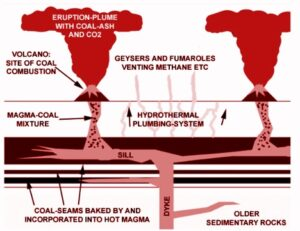January 28, 2023

# GravityGravity, also called Gravitation, in mechanics, the universal force of attraction acting between all matter. It is by far the weakest known force in nature and thus plays no role in determining the internal properties of everyday matter.

This force controls all the systems of the universe such as it controls our solar system, movement of all Stars, Galaxies and the whole cosmos. For the attraction of gravitational force all the matter clings to the earth’s surface.

All Human beings, chairs, tables, benches, Air, Water and all elements of our nature or environment cling on the earth’s surface by the attraction of gravitational force. gravity is responsible for keeping other objects in a stable position.

Nowadays, as the reason of the improvement of science we have invented many amazing things related to the gravitational force. Due to the force of gravity, water falls on the ground from the top of the hill. We can use this falling water to turn the turbine to generate electricity.

Due to the force of gravity, artificial satellites revolve around the earth. At the time of launching the rocket, this gravitational force has to be surpassed.

Therefore, we can use this force in different ways such as to track Earth’s water and ice, to provide energy, to give a boost to a spacecraft, use gravity as a telescope, to investigate unseen planets, to hunt for planets around other stars and at all to save the earth.

Gravity is the weakest of the four fundamental interactions of physics, approximately 1038 times weaker than the strong interaction, 1036 times weaker than the electromagnetic force  and 1029 times weaker than the weak interaction.

As a consequence, it has no significant influence at the level of subatomic particles. In contrast, it is the dominant interaction at the macroscopic scale, and is the cause of the formation, shape and trajectory (orbit) of astronomical bodies.

Gravity is measured by the gravitational acceleration which value is 9.8 ms-2, The Universal Gravitational Constant which value is 6.67*10-11 m3 kg-1 s-2, masses of two matter and the distance between them.

The Mathematical expression of Gravity or Gravitational force is –

Here F is the gravity or gravitational force, G is Gravitational Constant, m1 and m2 are the mass of two matter, r is the distance between these two matters, g is gravitational acceleration, m is the mass of any matter on the earth’s surface.

Mass is equivalent to the gravitational force. So as the mass increases, the force of gravity increases. Again, the force of gravity is proportional to the distance squire. So, if the distance increases, the force of gravity will decrease.

Gravity is one kind of Renewable Energy resource. It has no destruction. This is a blessing for our future generation. It will be possible to do a lot in the future.

#### You may have missed#### Causes of Permian Mass extinction